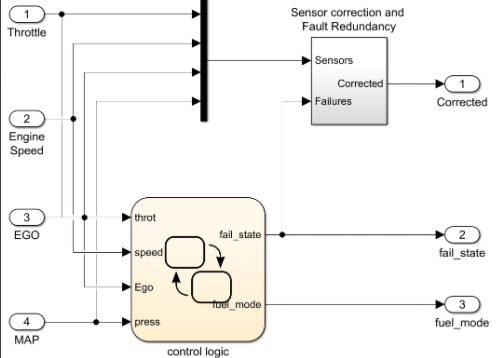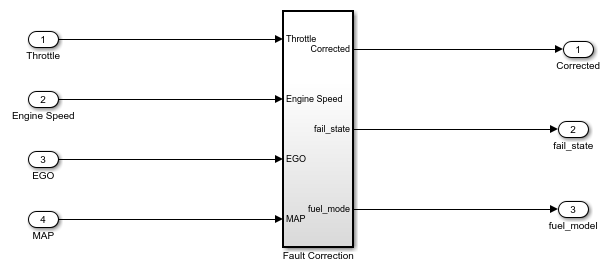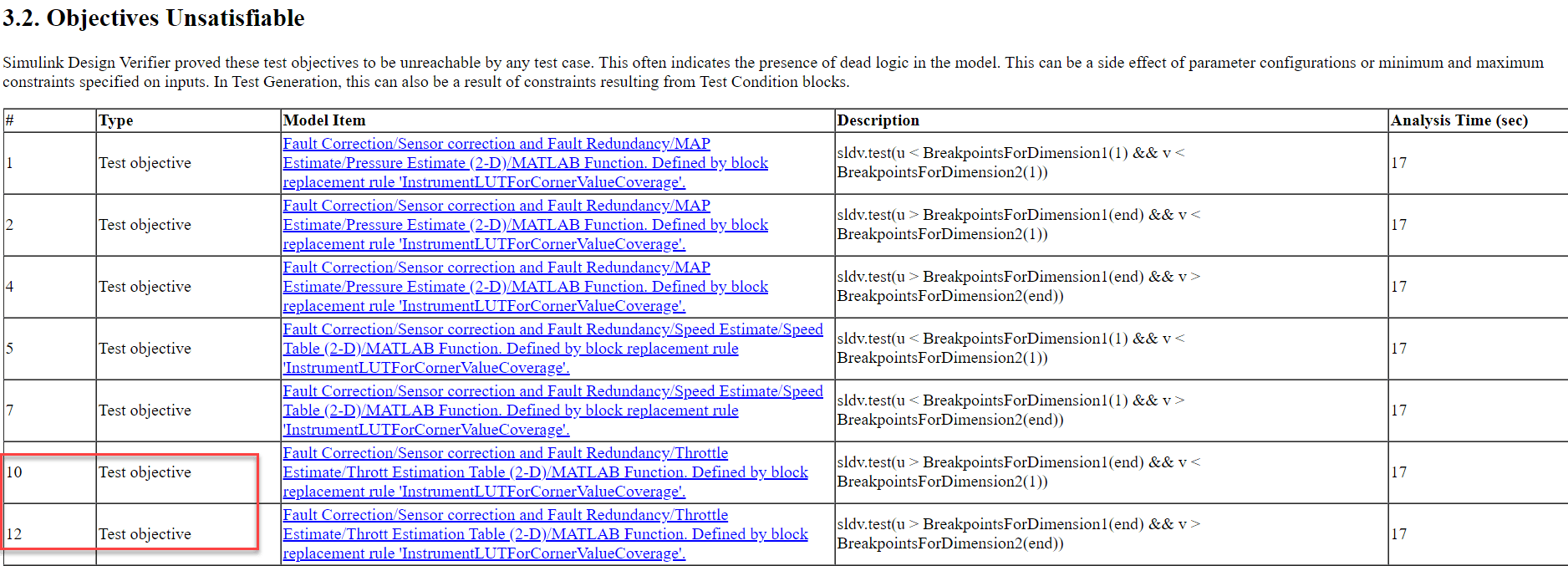## Analyze Coverage for Lookup Table Boundary Values

Lookup tables are standard block sets that approximate functions. Simulink® Coverage™ defines the coverage of lookup tables by checking if all grid points defined by breakpoints are covered or satisfied during simulation. For more information, see N-Dimensional Lookup Table (Simulink Coverage).

You can leverage Simulink Design Verifier™ to generate tests that hit the boundary values of a lookup table. The minimum and maximum breakpoints for each dimension, also known as the corner points, define the boundaries of the lookup table. Achieving such coverage is a common use case in the aerospace and automotive domains.

Consider this two-dimensional lookup table:The breakpoints that represent corner points are:

```Dimension 1 : 10, 30 Dimension 2: 1, 3```

The breakpoints that represent the boundaries of this lookup table are (10, 1), (10, 3), (30, 1), and (30,3). The tests corresponding to the lookup table boundary satisfy the following coverage metrics:

```Corner 1: Input 1 < 10, Input2 < 1 Corner 2: Input 1 < 10, Input2 > 3 Corner 3: Input 1 > 30, Input2 < 1 Corner 4: Input 1 > 30, Input2 > 3```

This example leverages block replacement framework provided by Simulink Design Verifier to generate tests.

Consider the controller unit (`control logic`) and the fault approximation unit (`Sensor correction and Fault Redundancy`) as shown:• `control logic`: The `control logic` statechart checks for the normal and failure modes of throttle, speed, and manifold absolute pressure (MAP).

• `Sensor correction and Fault Redundancy`: If there is a failure in the throttle, speed, and MAP values, the subsystem `Sensor correction and Fault Redundancy` approximates their values by using these tables:• `Throttle Estimate`: The lookup table ```Thrott Estimation Table (2-D)``` estimates the throttle position based on the values of speed and pressure.

• `Speed Estimate`: The lookup table ```Speed Table (2-D)``` estimates the speed based on the estimated value of throttle position and pressure.

• `MAP Estimate`: The lookup table ```Pressure Estimate (2-D)``` estimates the MAP based on the estimated value of speed and throttle.

Simulink Design Verifier generates boundary value coverage tests for each of these lookup tables. To describe the results, consider lookup table defined for ```Throttle Estimate``` as shown:• Throttle estimation lookup table uses speed (Sensor index '2') and manifold pressure (Sensor index '4') sensor values as inputs.

• The lookup table has 19 breakpoints for pressure with `0.05....0.95` as `corner points`, and 18 breakpoints for speed with `50....1e3` as ```corner points```.

The corner points for this lookup table are:

```Breakpoints 1 (speedVect): 50,1000 Breakpoints 2 (press): 0.05,0.95```

The generated tests attempt to hit the boundary points highlighted in the table. The tests require these breakpoint combinations to cover the boundary values.

Note

The `Engine Speed` and `MAP` inputs are inputs to the breakpoints `speedVect` and `press`, respectively.

```Corner 1: Engine Speed < 50, MAP < 0.05 Corner 2: Engine Speed < 50, MAP > 0.95 Corner 3: Engine Speed > 1000, MAP < 0.05 Corner 4: Engine Speed > 1000, MAP > 0.95```

The replacement rule, `InstrumentLUTForCornerValueCoverage` is shipped with the example mentioned here.

### Generate Tests for Lookup Table Boundary Values

This example shows how to generate tests for lookup table boundary value coverage.

Open the Model

```open_system('sldvdemo_fuelsys_lookup_corner_value_coverage'); ```Specify the Analysis Options

To specify the analysis options:

1. In the Apps tab, click Coverage Analyzer.

2. In the Coverage tab, click Settings to open the Configuration Parameters window.

3. Expand Other metrics, then select the Lookup table option. Click OK.

4. In the left pane, click Block Replacements. Select Apply block replacements.

5. In List of block replacement rules (in order of priority), specify the rule as `InstrumentLUTForCornerValueCoverage`. Click OK.

6. In the Test Generation pane, set Model Coverage Objectives to None.

Note: Because the test generation settings, Test conditions and Test Objectives are enabled, Simulink Design Verifier generates tests for the custom test objectives defined in the block replacement rule.

Perform Analysis

To generate tests only for the custom test objectives for the lookup table:

1. In the Design Verifier tab, click Generate Tests.

2. To view the coverage results, click the Simulate Tests and produce a model coverage report link.

Review Results

After simulating the test cases and generating a coverage report, the top left and right corners are covered while the bottom left and right corners are uncovered.The status of the test objectives associated with the 'Thrott Estimation Table (2-D)' Lookup table:

Status: Objectives SatisfiedFrom the above figure:

LUT Boundary: Engine Speed < 50, MAP < 0.05

Corner: Top Left

Analysis: This objectives is satisfied at time 0.03 (Step 4).

LUT Boundary: Engine Speed < 50, MAP > 0.95

Corner: Top Right

Analysis: This objectives is satisfied at time 0.21 (Step 22).

Status: Objectives UnsatisfiableFrom the above figure:

LUT Boundary: Engine Speed > 1000, MAP < 0.05

Corner: Bottom Left

Analysis: In the Control logic statechart, the input speed has the following values:

Minimum speed: 0 Maximum Speed: 1000

The statechart input speed is directly connected to the root inport Engine Speed. Hence, the same range constraints are applied to it. Due to these min/max constraints, the value of Engine Speed can never be set to a value >1000, hence this test objective becomes unsatisfiable.

LUT Boundary: Engine Speed > 1000, MAP > 0.95

Corner: Bottom Right

Analysis: The value of 'Engine Speed' can never be set to a value >1000, hence this test objective becomes unsatisfiable.

Clean Up

To complete the example, close the model.

```close_system('sldvdemo_fuelsys_lookup_corner_value_coverage',0); ```